﻿ 在材料力学教学中引入ABAQUS有限元数值分析的探讨 Discussion on the Introduction of ABAQUS Finite Element Analysis in Teaching Mechanics of Materials

Creative Education Studies
Vol. 07  No. 02 ( 2019 ), Article ID: 29757 , 6 pages
10.12677/CES.2019.72032

Discussion on the Introduction of ABAQUS Finite Element Analysis in Teaching Mechanics of Materials

Mingyao Li*, Jianping Zuo, Yingjie Li, Dongjie Xue, Dejun Liu

School of Mechanics & Civil Engineering, China University of Mining & Technology, Beijing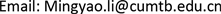Received: Mar. 26th, 2019; accepted: Apr. 10th, 2019; published: Apr. 17th, 2019ABSTRACT

The course of Mechanics of Materials involves some abstract concepts and obscure theories. It is noted in the teaching that most of students are generally aware of the difficulties of those concepts and theories. This paper proposes to introduce the ABAQUS finite element numerical analysis in the teaching of Mechanics of Materials. Taking the advantages of the dynamic visualization function, the interest of the students can be compelled, the understanding of the abstract concepts and theories can be deepened and the current situation of numerical methods less applied in traditional teaching can be improved. Therefore, the application of ABAQUS in the teaching of Mechanics of Materials is able to help improve the teaching effect and efficiency.

Keywords:Mechanics of Materials, Course Teaching, ABAQUS Finite Element Software1. 引言

2. 在材料力学教学中引入ABAQUS有限元数值分析的探讨

3. 教学案例——带孔平板的应力集中问题

3.1. 问题描述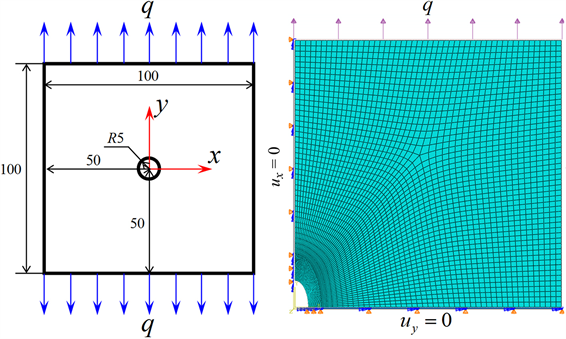(a) (b)

Figure 1. Model of the finite plates for stress concentration of hole; (a) Mechanical model; (b) Finite element model of ABAQUS

3.2. ABAQUS有限元数值分析

$x=0,\text{\hspace{0.17em}}{u}_{x}=0;\text{\hspace{0.17em}}y=0,\text{\hspace{0.17em}}{u}_{y}=0;\text{\hspace{0.17em}}x=\frac{L}{2},\text{\hspace{0.17em}}q=100\text{\hspace{0.17em}}\text{MPa};\text{\hspace{0.17em}}y=-\frac{L}{2},\text{\hspace{0.17em}}q=100\text{\hspace{0.17em}}\text{MPa}$ (1)

3.3. 应力集中现象的可视化

ABAQUS在后处理中的可视化(Visualization)模块中提供了应力、位移、应变等信息的云图。如图2(a)所示，受竖向拉载荷作用，加载方向的局部应力分布云图显示了孔边应力集中现象，即圆孔边左右两边集中了少量红色区域，其应力明显高于远离孔处的应力，最高值达到308.65 MPa，而远离孔边大部分区域的应力约为100 MPa。为了验证有限元计算的正确性，对比经典弹性力学理论对带孔的无限大平板中的应力解析解可知，在x轴方向上的环向正应力为：

${\sigma }_{\phi }=q\left(1+\frac{1}{2}\frac{{R}^{2}}{{\rho }^{2}}+\frac{3}{2}\frac{{R}^{4}}{{\rho }^{4}}\right)$ (2)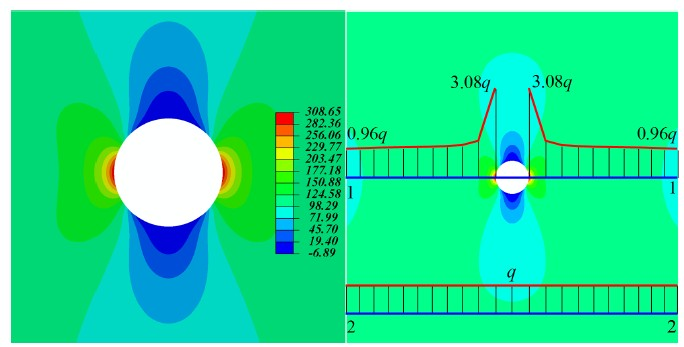(a) (b)

Figure 2. ABAQUS visualization of the stress concentration; (a) Local stress concentration nearby the hole; (b) Stress contour and stress distribution on sections

ABAQUS可视化功能清楚地显示整体结构的应力分布，在过圆孔中心x方向的1-1截面和几倍孔径外的2-2截面上分别画出相应的应力曲线，如图2(b)所示。在1-1截面上，孔边的应力远大于几倍孔径以外的应力，而应力随着据孔的距离增大而越快地趋近于无孔时的应力。在远离圆孔的2-2截面上，圆孔对应力分布的影响仅局限于孔的附近区域，在距离孔较远的地方应力几乎不受孔的影响，这就是圣维南原理。

3.4. 孔的形状对应力集中的影响分析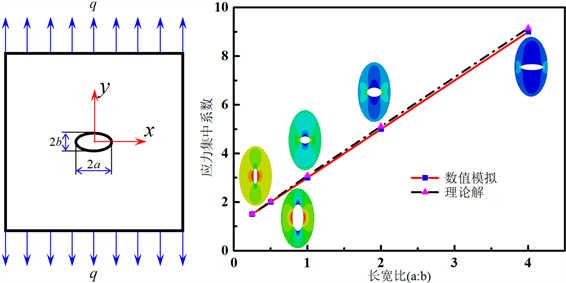(a) (b)

Figure 3. ABAQUS visualization of the stress concentration; (a) Mechanical model; (b) Comparison of theoretical and numerical results

3.5. 塑性屈服对应力集中的影响分析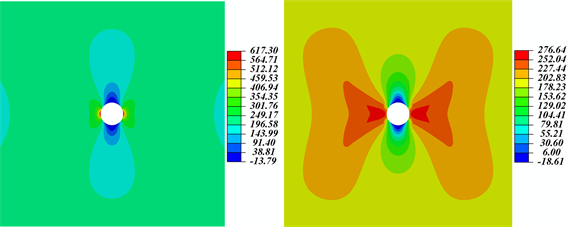(a) (b)

Figure 4. Effect of material property on stress concentration at hole-edge; (a) Elastic material; (b) Plastic material

4. 结论

Discussion on the Introduction of ABAQUS Finite Element Analysis in Teaching Mechanics of Materials[J]. 创新教育研究, 2019, 07(02): 178-183. https://doi.org/10.12677/CES.2019.72032

1. 1. 孔祥清, 翟城, 章文娇, 等. ABAQUS在材料力学教学中的应用展望[J]. 新校园旬刊, 2014(4): 22-22.

2. 2. 北京科技大学, 东北大学. 工程力学: 材料力学[M]. 北京: 高等教育出版社, 2008: 1.

3. 3. 徐芝纶. 弹性力学简明教程 [M]. 第五版. 北京: 高等教育出版社, 2016.

4. 4. 庄茁. 基于ABAQUS的有限元分析和应用[M]. 北京: 清华大学出版社, 2009.

5. 5. 谭林, 郭原. 开孔有限平板应力集中问题的有限元分析[J]. 重庆理工大学学报, 2015, 29(7): 35-39.